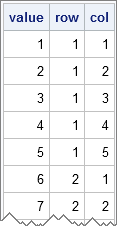If you write an n x p matrix from PROC IML to a SAS data set, you'll get a data set with n rows and p columns. For some applications, it is more convenient to write the matrix in a "long format" with np observations and three columns. The first column contains the cell values, the second column contains row indices, and the third column contains column indices.

It is easy to transform a rectangular matrix into "long form," so if you want to try it out yourself, stop reading now.

Given a matrix X, I have previously blogged about how to generate a matrix that is the same size as X, but that contains integer values that correspond to the rows (columns). The following SAS/IML module converts a matrix into a three-column matrix by using the COLVEC function. The CREATE and APPEND statements then write that data to a SAS data set:

```proc iml; start LongForm(X); /* convert numerical matrix to long format */ R = repeat(T(1:nrow(X)), 1, ncol(X)); /* row index */ C = repeat(1:ncol(X), nrow(X)); /* col index */ return( colvec(X) || colvec(R) || colvec(C) ); finish;   R = shape(1:25, 5, 5); /* initial 5 x 5 matrix */ S = LongForm(R); /* 25 x 3 matrix */ create Long from S[c={"value" "row" "col"}]; append from S; quit;   proc print data=Long nobs; run;```I will use this technique in a future blog post.

ShareDistinguished Researcher in Computational Statistics

Rick Wicklin, PhD, is a distinguished researcher in computational statistics at SAS and is a principal developer of PROC IML and SAS/IML Studio. His areas of expertise include computational statistics, simulation, statistical graphics, and modern methods in statistical data analysis. Rick is author of the books Statistical Programming with SAS/IML Software and Simulating Data with SAS.

1.Should the SPARSE function get a mention here? If you are worried about having zeros, then a quantity could be added to R beforehand to make it all positive and then the same amount taken it away form the first column of S afterwards.

•Yes! I originally had a paragraph about the SPARSE function, but removed it because, as you say, zero cells in the matrix are not included in the "long form." However, for matrices without zeros, the SPARSE function provides a built-in alternative.

•If you could be certain that the matrix does not contain any missing values, then the SPARSE function could be used (even when zeros are present) as follows:
.
S = sparse( choose(R, R, .) );
S = choose(S, S, 0);
.